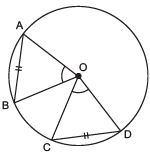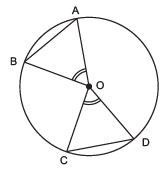Courses

# Ex 10.2 NCERT Solutions- Circles Class 9 Notes | EduRev

## Mathematics (Maths) Class 9

Created by: Full Circle

## Class 9 : Ex 10.2 NCERT Solutions- Circles Class 9 Notes | EduRev

The document Ex 10.2 NCERT Solutions- Circles Class 9 Notes | EduRev is a part of the Class 9 Course Mathematics (Maths) Class 9.
All you need of Class 9 at this link: Class 9

Question 1. Recall that two circles are congruent if they have the same radii. Prove that equal chords of congruent circles subtend equal angles at their centres.
Solution:
We have a circle having its centre at O and two equal chords AB and CD such that they subtend ∠AOB and ∠COD respectively at the centre, i.e. at O.
We have to prove that ∠AOB = ∠COD
Now, in ΔAOB and ΔCOD, we haveAO = CO               [Radii of the same circle]
BO = DO               [Radii of the same circle]
AB = CD               [Given]
∴ ΔAOB ≌ ΔCOD              [SSS criterion]
⇒ Their corresponding parts are equal.
∴ ∠AOB = ∠COD

Question 2. Prove that if chords of congruent circles subtend equal angles at their centres, then the chords are equal.
Solution:
We have a circle having its centre at O, and its two chords AB and CD such that
∠AOB = ∠COD
We have to prove that AB = CD∵ In ΔAOB and ΔCOD, we have:
AO = CO               [Radii of the same circle]
BO = DO               [Radii of the same circle]
∠AOB = ∠COD               [Given]
∴ ΔAOB ≌ ΔCOD              [SAS criterion]
∴ Their corresponding parts are equal, i.e. AB = CD

PERPENDICULAR FROM THE CENTRE TO A CHORD

REMEMBER

• The perpendicular from the centre of a circle to a chord bisects the chord.
• The line drawn through the centre of a circle to bisect a chord is perpendicular to the chord.
• There is one and only one circle passing through three given non-collinear points.

,

,

,

,

,

,

,

,

,

,

,

,

,

,

,

,

,

,

,

,

,

;